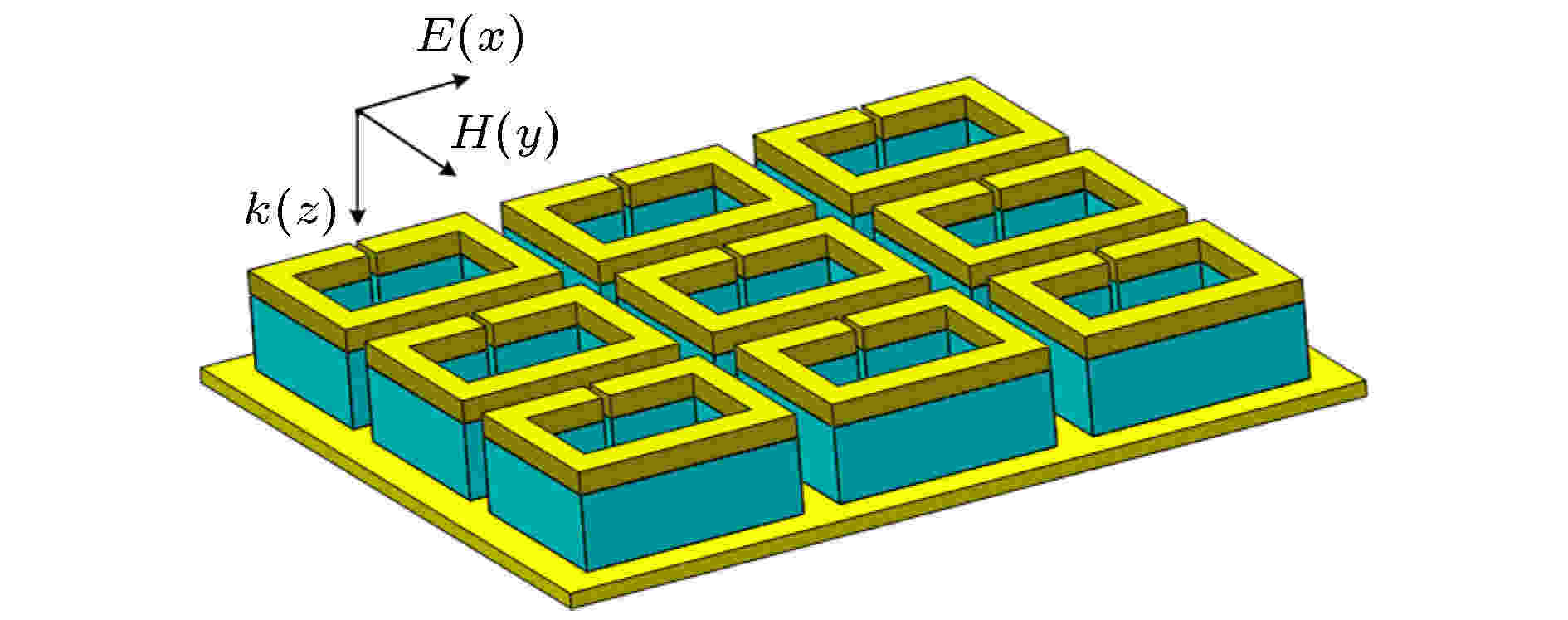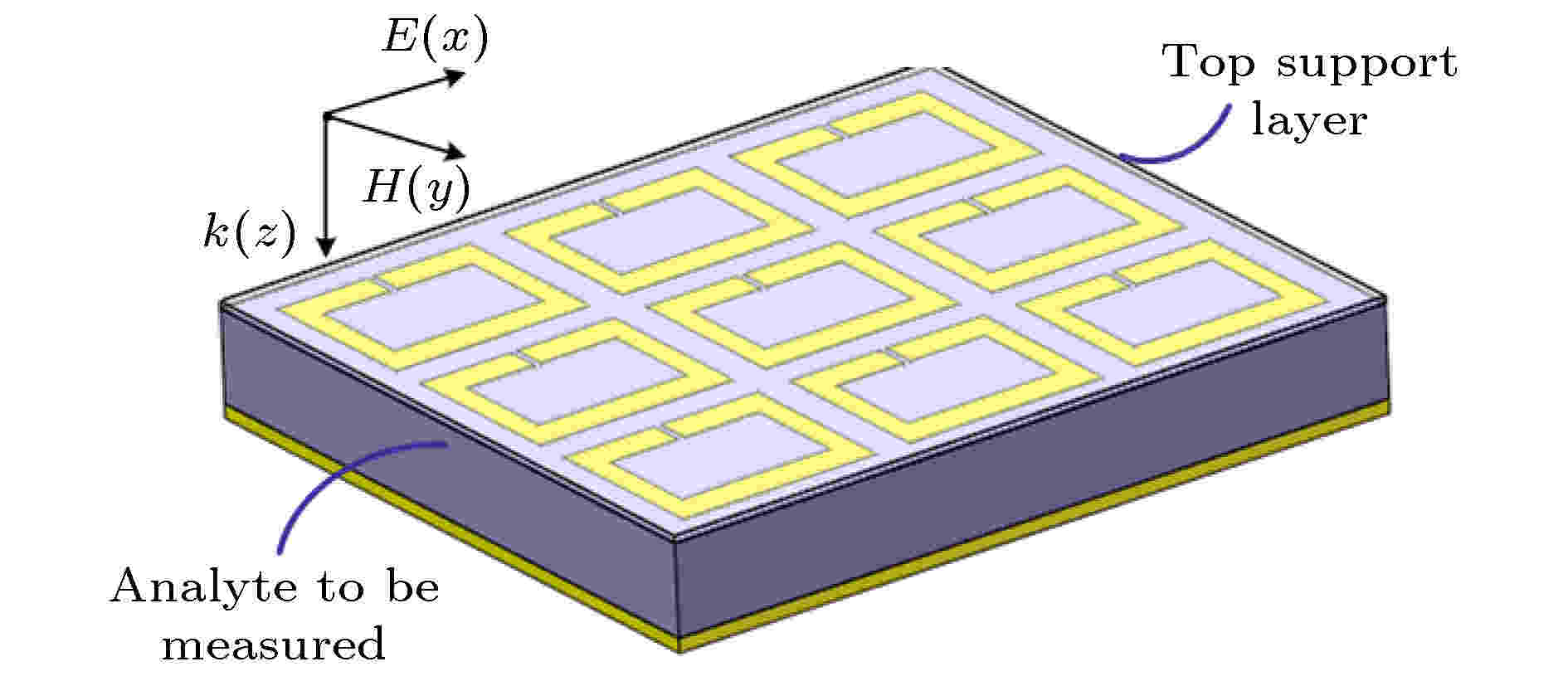#### 搜索

x## 留言板## 太赫兹波段电磁超材料吸波器折射率传感特性

PDF
HTML
• #### 摘要

太赫兹超材料吸波器作为一类重要的超材料功能器件, 除了可以实现对入射太赫兹波的完美吸收外, 还可以作为折射率传感器实现对周围环境信息变化的捕捉与监测. 通常从优化表面金属谐振单元结构和改变介质层材料和形态两个方面出发, 改善太赫兹超材料吸波器的传感特性. 为深入研究中间介质层对太赫兹超材料吸波器传感特性的影响, 本文基于金属开口谐振环阵列设计实现了具有连续介质层、非连续介质层和微腔结构的3款太赫兹超材料吸波器, 并对其传感特性与传感机理进行了深入研究. 结果表明, 为了提高太赫兹超材料吸波器的折射率灵敏度、最大探测范围等传感特性, 除了可以选用相对介电常数较小的材料作为中间介质层外, 还可以改变中间介质层的形态, 进而减小中间介质层对谐振场的束缚, 增强谐振场与被测分析物之间的耦合. 与传统的具有连续介质层的太赫兹超材料吸波器相比, 具有非连续介质层和微腔结构的超材料吸波器具有更优越的传感特性, 可应用于对待测分析物的高灵敏度、快速检测, 在未来的传感领域具有更加广阔的应用前景.

#### 作者及机构信息

###### 通信作者: 王俊林, wangjunlin@imu.edu.cn
• 基金项目: 国家自然科学基金(批准号: 51965047)、内蒙古自然科学基金(批准号: 2018MS06007)和内蒙古大学 2018 年高层次人才引进科研启动项目(批准号: 21700-5185128, 21700-5185131)资助的课题.

#### 参考文献

  Lee Y K 2012 太赫兹科学与技术原理 (北京: 国防工业出版社) 第1−30页 Lee Y K 2012 Principles of Terahertz Science and Technology (Beijing: National Defense Industry Press) pp1−30 (in Chinese)  Zhang X C, Alexander S, Zhang Y 2017 Nat. Photonics 11 16Zhang X C, Xu J Z 2010 Introduction to THz Wave Photonics (New York: Springer US) pp1−26  张活 2018 博士学位论文 (西安: 西安电子科技大学) Zhang H 2018 Ph. D. Dissertation (Xi’an: Xidian University) (in Chinese)  Wang J, Wang S, Singh R 2013 Chin. Opt. Lett. 11 011602Wang X, Zhang B Z, Wang W J, Wang J L, Duan J P 2017 IEEE Photonics J. 9 4600512黄文媛 2013 硕士学位论文 (成都: 西南交通大学) Huang W Y 2013 M. S. Dissertation (Chengdu: Southwest Jiaotong University) (in Chinese)  Li S Y, Ai X C, Wu R H 2018 Opt. Commun. 428 251闫昕, 张兴坊, 梁兰菊, 姚建铨 2014 光谱学与光谱分析 2365Yan X, Zhang X F, Liang L J, Yao J Q 2014 Spectrosc. Spect. Anal. 2365Chen T, Li S, Sun H 2012 Sensors 12 2742张玉萍, 李彤彤, 吕欢欢 2015 物理学报 64 117801Zhang Y P, Li T T, Lv H H 2015 Acta Phys. Sin. 64 117801Wang X, Zhang B Z, Wang W J, Duan J P 2017 IEEE Photonics J. 9 4600213毛前军, 冯春早 2019 光学学报 39 0816001Mao Q J, Feng C Z 2019 Acta Opt. Sin. 39 0816001Wang W, Yan F P, Tan S Y 2017 Photonics Res. 5 571Yan X, Yang M S, Zhang Z 2019 Biosens. Bioelectron. 126 485Srivastava Y K, Cong L Q, Singh R 2017 Appl. Phys. Lett. 111 201101Ahmed S, Sungjoon L 2018 Biosens. Bioelectron. 117 398Han B J, Han Z H, Qin J Y 2019 Talanta 192 1Singh R, Al-Naib A I, Koch M 2010 Opt. Express 18 13044Saraswati B, Kyoungsik K 2019 J. Phys. D: Appl. Phys. 52 275106Li W Y, Su Y, Zhai X 2018 IEEE Photonic. Tech. Lett. 30 2068Shen S M, Liu Y L, Liu W Q 2018 Mater. Res. Express 5 125804Hu T, Strikwerda A C, Liu M 2010 Appl. Phys. Lett. 97 261909Moritake Y, Tanaka T 2018 Opt. Express 26 3674Brian B, Sepúlveda B, Alaverdyan Y, Lechuga L M, Käll M 2009 Opt. Express 17 2015Wang W, Yan F P, Tan S Y 2020 Photonics Res. 8 519Meng K, Park S J, Burnett A D 2019 Opt. Express 27 23164Hu T, Chieffo L R, Brenckle M A, et al. 2016 Adv. Mater. 23 3197Dmitriev A, Hägglund C, Chen S 2008 Nano Lett. 8 3893Whitesides G M 2006 Nature 442 368Zhou H, Hu D L, Yang C 2018 Sci. Rep. 8 14801Hu X, Xu G Q, Wen L 2016 Laser Photonics Rev. 10 962Janneh M, De Marcellis A, Palange E 2018 Opt. Commun. 416 152Wang B X, Zhai X, Wang G Z 2015 Appl. Phys. 117 014504#### 施引文献

• 图 1  基于连续介质层和金属开口谐振环阵列的太赫兹超材料吸波器的结构示意图

Fig. 1.  Schematic diagram of THz MM absorber based on continuous dielectric layer and metallic split-ring resonator array.

图 2  具有连续介质层的太赫兹超材料吸波器的吸收特性仿真曲线

Fig. 2.  Simulated absorption characteristic curve of THz MM absorber with continuous dielectric layer.

图 3  (a) 谐振频率处的表面电流分布; (b) 谐振频率处的表面电场分布

Fig. 3.  (a) Surface current distribution at the resonance frequency; (b) surface electric field distribution at the resonance frequency.

图 4  (a) 谐振频率处$y$= 0 截面的电场分布; (b) 谐振频率处$x$= 0 截面的磁场分布

Fig. 4.  (a) Electric field distribution at cross section of $y$= 0 at the resonance frequency; (b) magnetic field distribution at cross section of $x$= 0 at the resonance frequency

图 5  在分析物折射率从n = 1变化到n = 1.8时具有连续介质层的太赫兹超材料吸波器的吸收特性仿真曲线

Fig. 5.  Simulated absorption characteristic curves of THz MM absorber with continuous dielectric layer under analyte refractive index changes from n = 1 to n = 1.8.

图 6  在分析物折射率从n = 1变化到n = 1.8时具有连续介质层的太赫兹超材料吸波器的谐振频率偏移及其线性拟合

Fig. 6.  Resonance frequency shifts and linear fitting of THz MM absorber with continuous dielectric layer under analyte refractive index changes from n = 1 to n = 1.8.

图 7  介质层材料的相对介电常数变化对传感器折射率频率灵敏度的影响

Fig. 7.  Influence of relative permittivity of dielectric layer material on the refractive index frequency sensitivity of the sensor.

图 8  在分析物厚度不同条件下, 分析物折射率从n = 1变化到n = 1.8时具有连续介质层的太赫兹超材料吸波器的谐振频率偏移

Fig. 8.  Resonance frequency shifts of THz MM absorber with continuous dielectric layer under analyte refractive index changes from n = 1 to n = 1.8 for different thicknesses of the analyte.

图 9  选用连续介质层的太赫兹超材料吸波器作为传感器时, 被测分析物厚度对传感器折射率频率灵敏度的影响

Fig. 9.  Influence of the thickness of the analyte to be measured on the refractive index frequency sensitivity of the sensor for the THz MM absorber with continuous dielectric layer.

图 10  基于非连续介质层和金属开口谐振环阵列的太赫兹超材料吸波器的结构示意图

Fig. 10.  Schematic diagram of THz MM absorber based on discontinuous dielectric layer and metallic split-ring resonator array.

图 11  具有非连续介质层的太赫兹超材料吸波器的吸收特性仿真曲线

Fig. 11.  Simulated absorption characteristic curve of THz MM absorber with discontinuous dielectric layer.

图 12  在分析物折射率从n = 1变化到n = 1.8时具有非连续介质层的太赫兹超材料吸波器的吸收特性仿真曲线

Fig. 12.  Simulated absorption characteristic curves of THz MM absorber with discontinuous dielectric layer under analyte refractive index changes from n = 1 to n = 1.8.

图 13  在分析物折射率从n = 1变化到n = 1.8时具有非连续介质层的太赫兹超材料吸波器的谐振频率偏移及其线性拟合

Fig. 13.  Resonance frequency shifts and linear fitting of THz MM absorber with discontinuous dielectric layer under analyte refractive index changes from n = 1 to n = 1.8.

图 14  在分析物厚度不同条件下, 分析物折射率从n = 1变化到n = 1.8时具有非连续介质层的太赫兹超材料吸波器的谐振频率偏移

Fig. 14.  Resonance frequency shifts of THz MM absorber with discontinuous dielectric layer under analyte refractive index changes from n = 1 to n = 1.8 for different thicknesses of the analyte.

图 15  选用非连续介质层的太赫兹超材料吸波器作为传感器时, 被测分析物厚度对传感器折射率频率灵敏度的影响

Fig. 15.  Influence of the thickness of the analyte to be measured on the refractive index frequency sensitivity of the sensor for the THz MM absorber with discontinuous dielectric layer.

图 16  待测分析物充当介质层的太赫兹超材料吸波器的结构示意图

Fig. 16.  Schematic diagram of THz MM absorber whose analyte to be measured acts as dielectric layer.

图 17  未填充待测分析物的太赫兹超材料吸波器的吸收特性仿真曲线

Fig. 17.  Simulated absorption characteristic curve of THz MM absorber without filling the analyte to be measured.

图 18  分析物折射率从n = 1变化到n=1.8时具有微腔结构的太赫兹超材料吸波器的吸收特性仿真曲线

Fig. 18.  Simulated absorption characteristic curve of THz MM absorber with microcavity structure under analyte refractive index range from n = 1 to n = 1.8.

图 19  分析物折射率从n = 1变化到n = 1.8时具有微腔结构的太赫兹超材料吸波器的谐振频率偏移及其线性拟合

Fig. 19.  Resonance frequency shifts and linear fitting of THz MM absorber with microcavity structure under analyte refractive index changes from n = 1 to n = 1.8.

表 1  太赫兹超材料吸波器的参数对比

Table 1.  Comparison of parameters of THz MM absorbers

 太赫兹超材料吸波器的吸收与传感特性参数 具有不同介质层的太赫兹超材料吸波器 连续介质层 非连续介质层 微腔结构 谐振频率/THz 0.183 0.245 0.277 吸收率/% 99.97 93.30 86.60 谐振峰半高宽FWHM/GHz 9.3 13.0 15.0 品质因数Q 19.7 18.8 18.4 折射率灵敏度S/(GHz·RIU–1) 8.6 65.8 101.5 FOM值 0.92 5.06 6.77
•  引用本文:Citation:## 太赫兹波段电磁超材料吸波器折射率传感特性

• 内蒙古大学电子信息工程学院, 呼和浩特　010021
• ###### 通信作者: 王俊林, wangjunlin@imu.edu.cn
基金项目: 国家自然科学基金(批准号: 51965047)、内蒙古自然科学基金(批准号: 2018MS06007)和内蒙古大学 2018 年高层次人才引进科研启动项目(批准号: 21700-5185128, 21700-5185131)资助的课题.

### English Abstract2.   具有连续介质层的太赫兹超材料吸波器3.   具有非连续介质层的太赫兹超材料吸波器4.   具有微腔结构的太赫兹超材料吸波器

PDF查看关注分享

### 目录/下载:  全尺寸图片 幻灯片